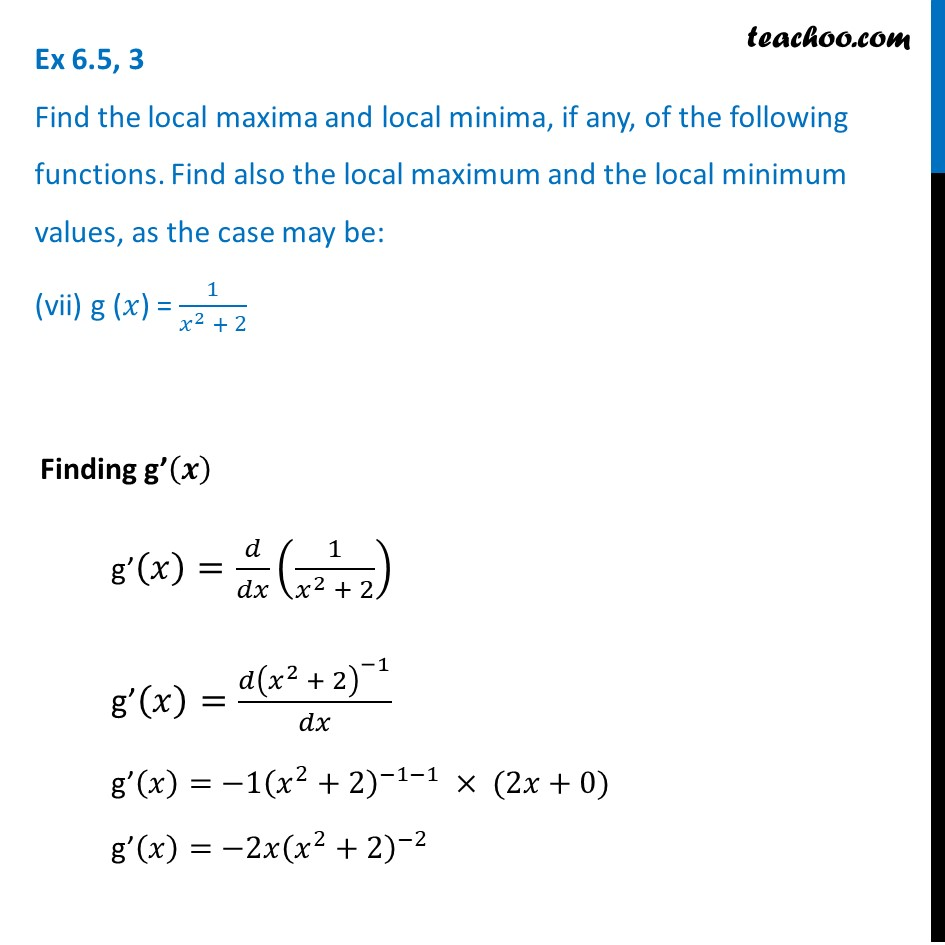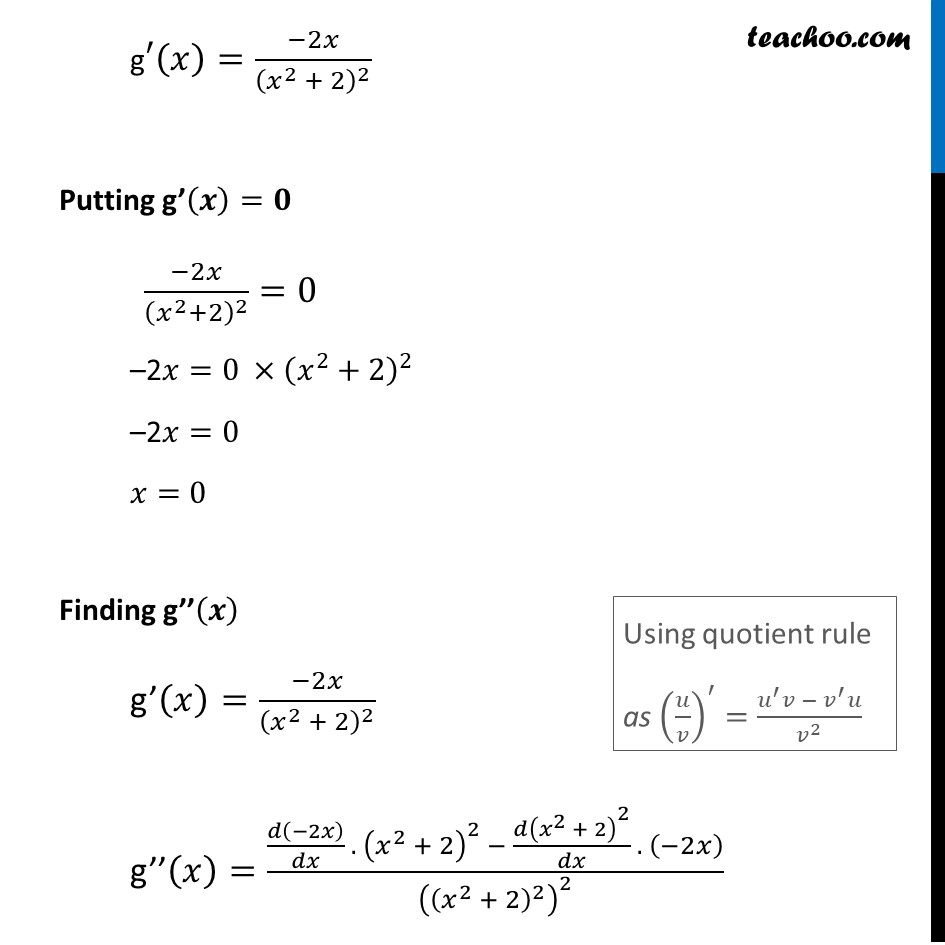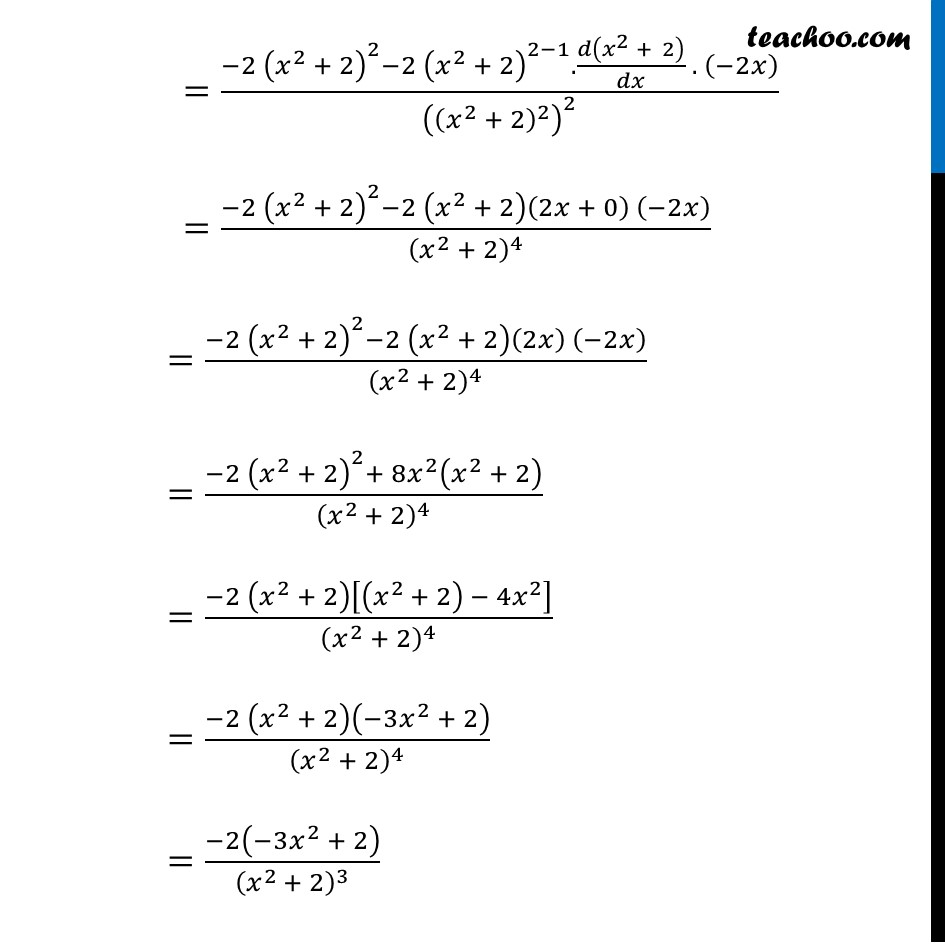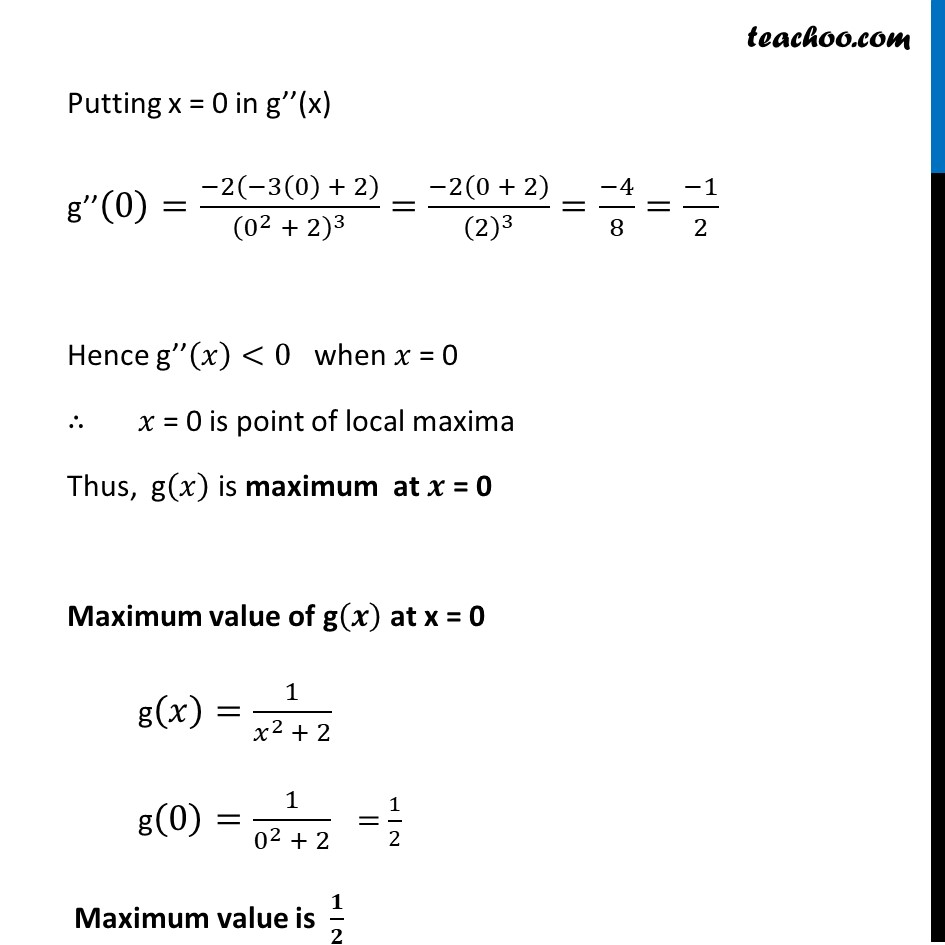Ex 6.5

Chapter 6 Class 12 Application of Derivatives
Serial order wiseMaths Crash Course - Live lectures + all videos + Real time Doubt solving!

### Transcript

Ex 6.5, 3 Find the local maxima and local minima, if any, of the following functions. Find also the local maximum and the local minimum values, as the case may be: (vii) g (𝑥) = 1/(𝑥^2 + 2)Finding g’(𝒙) g’(𝑥)=𝑑/𝑑𝑥 (1/(𝑥^2 + 2)) g’(𝑥)=(𝑑(𝑥^2 + 2)^(−1))/𝑑𝑥 g’(𝑥)=−1(𝑥^2+2)^(−1−1) × (2𝑥+0) g’(𝑥)=−2𝑥(𝑥^2+2)^(−2) g′(𝑥)=( −2𝑥 )/(𝑥^2 + 2)^2 Putting g’(𝒙)=𝟎 ( −2𝑥 )/(𝑥^2+2)^2 =0 –2𝑥=0 ×(𝑥^2+2)^2 –2𝑥=0 𝑥=0 Finding g’’(𝒙) g’(𝑥)=(−2𝑥)/(𝑥^2 + 2)^2 g’’(𝑥)=(𝑑(−2𝑥)/𝑑𝑥 . 〖 (𝑥^2 + 2)〗^2 − (𝑑(𝑥^2 + 2)^2)/𝑑𝑥 . (−2𝑥))/((𝑥^2 + 2)^2 )^2 Using quotient rule as (𝑢/𝑣)^′=(𝑢^′ 𝑣 − 𝑣^′ 𝑢)/𝑣^2 =(−2 (𝑥^2 + 2)^2−2 (𝑥^2 + 2)^(2−1).𝑑(𝑥^2 + 2)/𝑑𝑥 . (−2𝑥))/((𝑥^2 + 2)^2 )^2 =(−2 (𝑥^2 + 2)^2−2 (𝑥^2 + 2)(2𝑥 + 0) (−2𝑥))/(𝑥^2 + 2)^4 =(−2 (𝑥^2 + 2)^2−2 (𝑥^2 + 2)(2𝑥) (−2𝑥))/(𝑥^(2 )+ 2)^4 =(−2 (𝑥^2 + 2)^2+ 8𝑥^2 (𝑥^2 + 2))/(𝑥^(2 )+ 2)^4 =(−2 (𝑥^2 + 2)[(𝑥^(2 )+ 2) − 4𝑥^2 ])/(𝑥^2 + 2)^4 =(−2 (𝑥^2 + 2)(−3𝑥^2 + 2))/(𝑥^2 + 2)^4 =(−2(−3𝑥^2 + 2))/(𝑥^(2 )+ 2)^3 Putting x = 0 in g’’(x) g’’(0)=(−2(−3(0) + 2))/(0^2 + 2)^3 =(−2(0 + 2))/(2)^3 =(−4)/8=(−1)/2 Hence g’’(𝑥)<0 when 𝑥 = 0 ∴ 𝑥 = 0 is point of local maxima Thus, g(𝑥) is maximum at 𝒙 = 0 Maximum value of g(𝒙) at x = 0 g(𝑥)=1/(𝑥^(2 )+ 2) g(0)=1/(0^2 + 2) = 1/2 Maximum value is 𝟏/𝟐